Next: Finding optimal for Dirichlet Up: Optimising the cost function Previous: Optimising the cost function   Contents

### Finding optimal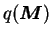Assuming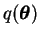is fixed, the cost function can be written, up to an additive constant, in the form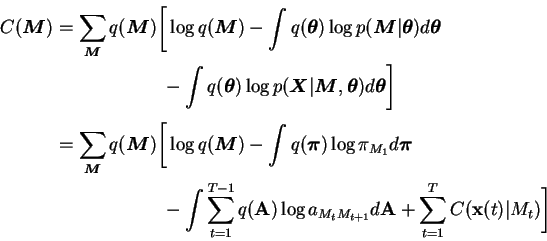(6.11)

where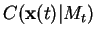is the value of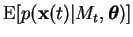, i.e. the cost'' of current data sample given the HMM state.

By defining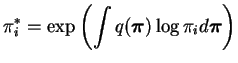and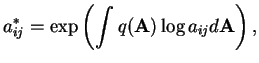(6.12)

Equation (6.11) can be written in the form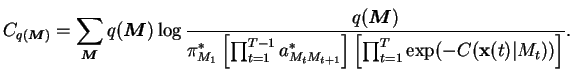(6.13)

The expression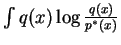is minimised with respect to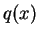by setting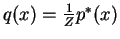where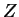is the appropriate normalising constant . This can be proved with similar reasoning as in Equation (3.13).

The cost in Equation (6.13) can thus be minimised by setting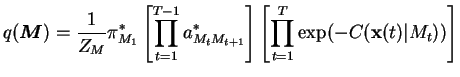(6.14)

where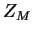is the appropriate normalising constant.

The derived optimal approximation is very similar in form to the exact posterior in Equation (4.6). Therefore the point probabilities of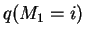and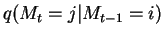can be evaluated with a modified forward-backward iteration. The result is the same as in Equation (4.9) except that in the iteration,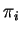is replaced with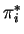,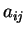is replaced with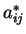and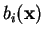is replaced with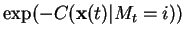.Next: Finding optimal for Dirichlet Up: Optimising the cost function Previous: Optimising the cost function   Contents
Antti Honkela 2001-05-30